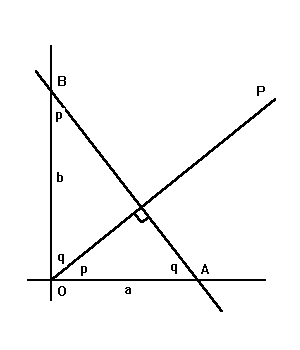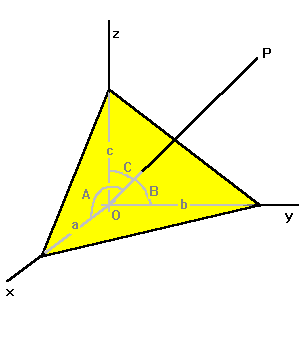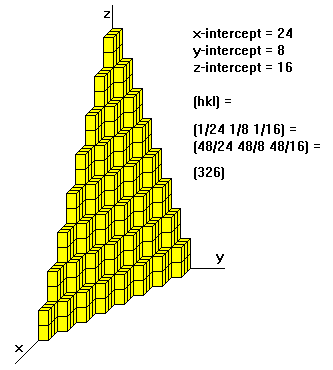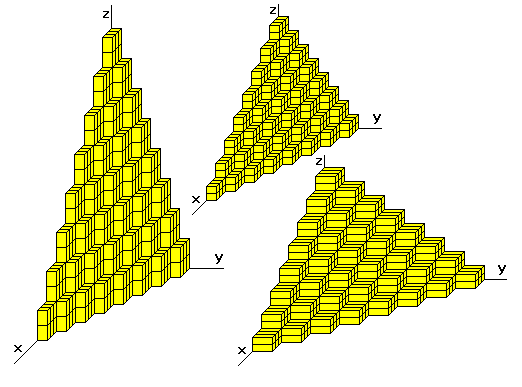Miller Indices

Steven Dutch, Natural and Applied Sciences, University of Wisconsin - Green Bay
First-time Visitors: Please visit Site Map and Disclaimer. Use "Back" to return here.

Lines in the PlaneWhat are the equations of lines AB and OP?. The generic equation of a line is y = mx + b, where b is the y-intercept and m is the slope. For line AB, the slope is -tan q, or -b/a. Thus we have:Line AB: y = -bx/a + b, or, x/a + y/b = 1. If OP is perpendicular to AB, its y- intercept is obviously zero, and we have y = x tan p, or y = xa/b, or xa = yb. Thus, a general line has a simple equation in terms of its intercepts, and a line through the origin perpendicular to the line also has a simple equation in terms of the intercepts. As a general rule, if two lines are perpendicular, the product of their slopes is -1.

Lines and Planes in Three DimensionsThe equation for a plane in three dimensions is exactly analogous for the line in two dimensions: x/a + y/b + z/c = 1. If line OP is perpendicular to the plane, its equation is also analogous to the two-dimensional case: xa = yb = zc. If we know a plane, we know the line through the origin perpendicular to it, called the pole to the plane. Quantities a, b and c are termed the direction numbers of the line. Line OP makes angles A, B, and C with the axes. The angles are related as follows: cos2 A + cos2 B + cos2 C = 1

Cos A, cos B and cos C are called the direction cosines of the line. They are obviously also direction numbers. If you know any arbitrary direction numbers a, b and c, then cos A = a/s, cos B = b/s and cos c = c/s, where s2 = a2 + b2 + c2

Miller Indices

Now that we know the equation of a plane in space, the rules for Miller Indices are a little more intelligible. They are:

• Determine the intercepts of the face along the crystallographic axes, in terms of unit cell dimensions.
• Take the reciprocals
• Clear fractions
• Reduce to lowest terms

For example, if the x-, y-, and z- intercepts are 2,1, and 3, the Miller indices are calculated as:

• Take reciprocals: 1/2, 1/1, 1/3
• Clear fractions (multiply by 6): 3, 6, 2
• Reduce to lowest terms (already there)

Thus, the Miller indices are 3,6,2. If a plane is parallel to an axis, its intercept is at infinity and its Miller index is zero. A generic Miller index is denoted by (hkl).If a plane has negative intercept, the negative number is denoted by a bar above the number. Never alter negative numbers. For example, do not divide -1, -1, -1 by -1 to get 1,1,1. This implies symmetry that the crystal may not have!

For hexagonal and trigonal minerals, there are three possible axis directions, spaced 120 degrees apart:

+x2\           /-x3
\         /
\       /
\     /
\   /
-x1            \ /             +x1
----------------+----------------
/ \
/   \
/     \
/       \
/         \
+x3/           \-x2

Obviously, any two intercepts specify the face. Also, there will be two intercepts of one sign and one of the other. The Miller indices for a hexagonal mineral are often written hikl. Indices h, i and k are related by h + i + k = 0. Some modern texts dispense with the i term and treat hexagonal minerals like all others.

Some General Principles

• If a Miller index is zero, the plane is parallel to that axis.
• The smaller a Miller index, the more nearly parallel the plane is to the axis.
• The larger a Miller index, the more nearly perpendicular a plane is to that axis.
• Multiplying or dividing a Miller index by a constant has no effect on the orientation of the plane
• Miller indices are almost always small.

Why Miller Indices?

• Using reciprocals spares us the complication of infinite intercepts.
• Formulas involving Miller indices are very similar to related formulas from analytical geometry.
• Specifying dimensions in unit cell terms means that the same label can be applied to any face with a similar stacking pattern, regardless of the crystal class of the crystal. Face 111 always steps the same way whether the crystal is isometric or triclinic.Miller Indices and Lines

The Miller Index of a line is about as simple as it can be: if the line passes through (h, k, l), its Miller Index is [hkl], written in brackets to distinguish it from a face.

Zones

A family of faces all parallel to some particular line is called a zone, and the line is called the zone axis. Two faces (hkl) and (pqr) belong to zone [kr-lq, lp-hr, hq-kp]. Note the similarity of this formula to the cross-product formula from vector mechanics. Any other face (def) belongs to the same zone if its indices are some linear combination of (hkl) and (pqr), for example, d = 2h-3p, e = 2k-3q, etc.

For example, faces (110) and (010) belong to [1*0-0*1, 0*0-1*0, 1*1-1*0], or . The final zero in the face indices is a tipoff that they are both parallel to the z-axis, and the zone index  is the z-axis. Any other face whose index is some linear combination of (110) and (010) is also a member of that zone. Obviously the final index must be zero.

What about faces (211) and (124)? Their zone axis is [1*4-1*2, 1*1-2*4, 2*2-1*1] or [2,-7,1]. Faces (335), (546), (1,-1,-3), etc. also belong to this zone.